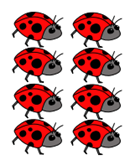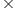Mathematics
Easy

Question

# Choose the equation which describes the array.## 3 + 3 = 6    2 + 2 + 2 = 6    4 + 4 = 8    3 + 3 + 3 = 9Hint:

## The correct answer is: 4 + 4 = 8

### An array is a group of object, pictures or numbers arranged in columns and rows.In the above image ,  Columns = 2 and 4insects in each columnThus, 4 + 4 = 8 , describes the array.

Alternatively,In the above image , Rows = 4 and Columns = 2
Thus, RowsColumns = 42 = 8elements
Thus, 4 + 4 = 8 , describes the array.

### Related Questions to study#### With Turito Foundation.#### Get an Expert Advice From Turito.